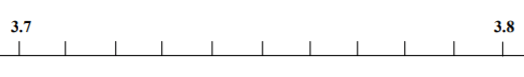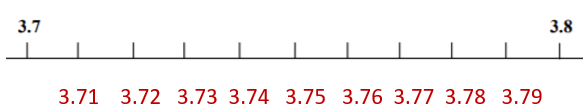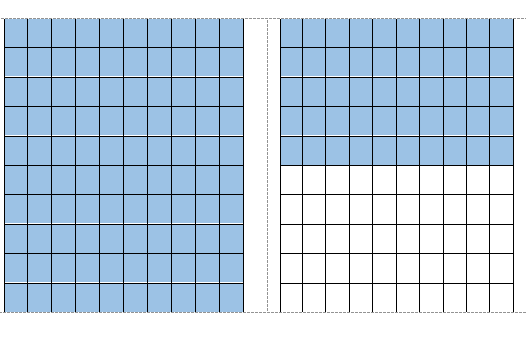# Math Worksheets Land

Math Worksheets For All Ages

# Math Worksheets Land

Math Worksheets For All Ages

Home > Math Topics > Decimals >

# Visualizing Decimals Worksheets

Decimal values allow students to highlight a value that is less than one and not as difficult to work with as fractions are. When visualizing decimals, we will only move over the course of two places tenths and hundredths. This is because drawing a model that illustrates the thousandths or ten-thousandths place would take an entire page of paper. We encourage students to use both the interval models and number lines that we illustrate here for you to improve your understanding and comfort level with values written in decimal format. These worksheets and lessons help students get a much better understanding of what a decimal value actually means.

### Aligned Standard: 5.NBT.3

• Answer Keys - These are for all the unlocked materials above.

### Homework Sheets

You will definitely need the help of some type of coloring tool (marker, crayon, etc.) for these.

• Homework 1 - You will actually see that 0.8 can be represented as eight of ten colored boxes.
• Homework 2 - Write the number the picture shows as a) a fraction and b) a decimal.
• Homework 3 - For number one, they need to count the number of rows and columns.

### Practice Worksheets

I always call these rush the grid activities. Kids really get a lot out of these.

• Practice 1 - Write the decimal represented by the grids.
• Practice 2 - Fill in the missing parts on each number line.
• Practice 3 - We introduce the concept of one whole unit in addition to a decimal here.

### Math Skill Quizzes

For some you will need to draw and for others you will need to conceptualize the drawings.

• Quiz 1 - Six problems to see if you know what you are talking about.
• Quiz 2 - This works off of the ten by ten grids.
• Quiz 3 - A mix of number lines and grid styles.

### How to Relate Drawings to Decimal Values

The concept of decimals is tough to understand, especially for kids who are just beginning to learn how you can represent math through drawings and sketches. Thus, there are different ways, and you can teach students about decimal numbers, which includes drawings as well.

Number Lines - Firstly, the concept of number lines is quite common when you want to show the children the concept of decimals. It's just as same as a ruler, but number lines can be more definite. When using them you just need to determine the start and finish and then how many intervals exist between them. Explore the sample below and see if you can label all the missing intervals:There are 10 intervals that exist between 3.7 and 3.8. This would indicate that every value differs by 0.01 (a hundredth). We can therefore label each of the places by increasing each interval by 0.01.Interval Boxes - Another way to use drawings for the concept of decimals is by using a bunch of boxes for it. We call these interval boxes, and they follow a very familiar flow to the number lines. We normal use boxes that are either broken into 10 parts (tenths) or 100 parts (hundredths). For instance, if you want to learn how many cents there are in \$1.50, then you can make two sets of 100 boxes. You can color one set completely, while you can color 50 boxes from the other set. It would appear like this:Unlock all the answers, worksheets, homework, tests and more!
Save Tons of Time! Make My Life Easier Now

## Thanks and Don't Forget To Tell Your Friends!

I would appreciate everyone letting me know if you find any errors. I'm getting a little older these days and my eyes are going. Please contact me, to let me know. I'll fix it ASAP.